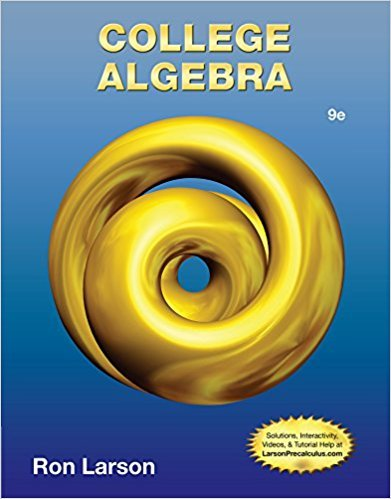×
×

# In Exercises 2146, is related to one of the parent functions | Ch 2 - 2.5.28, 9thISBN: 9781133963028 204

## Solution for problem 2.5.28 Chapter 2

College Algebra | 9th Edition

• Textbook Solutions
• 2901 Step-by-step solutions solved by professors and subject experts
• Get 24/7 help from StudySoup virtual teaching assistantsCollege Algebra | 9th Edition

4 5 1 309 Reviews
25
3
Problem 2.5.28

In Exercises 2146, is related to one of the parent functions described in Section 2.4. (a) Identify the parent function (b) Describe the sequence of transformations from to (c) Sketch the graph of (d) Use function notation to write in terms of f gx 14x 2gx2 x 52 2 2gx2x 72 g

Step-by-Step Solution:
Step 1 of 3
Step 2 of 3

Step 3 of 3

##### ISBN: 9781133963028

This textbook survival guide was created for the textbook: College Algebra, edition: 9. The answer to “In Exercises 2146, is related to one of the parent functions described in Section 2.4. (a) Identify the parent function (b) Describe the sequence of transformations from to (c) Sketch the graph of (d) Use function notation to write in terms of f gx 14x 2gx2 x 52 2 2gx2x 72 g” is broken down into a number of easy to follow steps, and 52 words. The full step-by-step solution to problem: 2.5.28 from chapter: 2 was answered by , our top Math solution expert on 01/02/18, 09:21PM. College Algebra was written by and is associated to the ISBN: 9781133963028. Since the solution to 2.5.28 from 2 chapter was answered, more than 231 students have viewed the full step-by-step answer. This full solution covers the following key subjects: . This expansive textbook survival guide covers 9 chapters, and 5750 solutions.

Unlock Textbook Solution